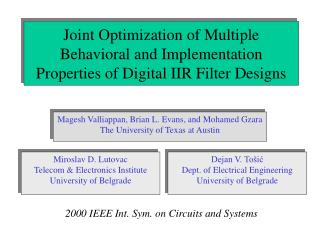DownloadDownload PresentationMagesh Valliappan, Brian L. Evans, and Mohamed Gzara The University of Texas at Austin

# Magesh Valliappan, Brian L. Evans, and Mohamed Gzara The University of Texas at Austin

Télécharger la présentation## Magesh Valliappan, Brian L. Evans, and Mohamed Gzara The University of Texas at Austin

- - - - - - - - - - - - - - - - - - - - - - - - - - - E N D - - - - - - - - - - - - - - - - - - - - - - - - - - -
##### Presentation Transcript

1. Joint Optimization of Multiple Behavioral and Implementation Properties of Digital IIR Filter Designs Magesh Valliappan, Brian L. Evans, and Mohamed Gzara The University of Texas at Austin Miroslav D. Lutovac Telecom & Electronics Institute University of Belgrade Dejan V. Tošić Dept. of Electrical Engineering University of Belgrade 2000 IEEE Int. Sym. on Circuits and Systems

2. Introduction • Problem • Simultaneously optimize multiple characteristics of an existing digital IIR lowpass filter design • Goal • Develop an extensible automated framework • Solution • Solve constrained nonlinear optimization problem by using sequential quadratic programming (SQP) • Program Mathematica to derive formulas and generate Matlab programs to perform optimization

3. Modeling • Free parameters • Set of n conjugate pole pairs akexp(± j bk) • Set of m conjugate zero pairs ciexp(± j di) • Properties • Behavioral: magnitude response, phase response • Implementation: quality factors • Compute • Cost function as a weighted mean of distance measures • Constraints to enforce numerical stability • Closed-form symbolic gradients for robustness

4. Objective Measures • Magnitude response • Scale to be unity at DC • Unwrapped phase response • Constrain zeros to be outside passband • Quality factor Q • For each pole pair ak exp(± j bk): 0.5  Qk  • Effective quality factor is geometric mean of Qk factors

5. Distance Measures • Non-negative differentiable measures • A value of zero means the ideal case • Differentiability necessary for SQP formulation • Deviation in magnitude response • L2 norm of deviation from ideal in passband, stopband and transition bands • Deviation of quality factors • Minimum effective quality factor Qeff is 0.5 • Deviation measured as sq = Qeff - 0.5

6. Distance Measures • Deviation from linear phase in passband • L2 norm of deviation from perfect linear phase at optimal slope within (0, l) where l p • Approximate optimal slope to obtain analytic form • Weighted mean of two first-order estimates • Accurate up to four Taylor series terms •  = 0.4163;  = 0.5837; r1= 0.5385; r2 = 0.9062

7. Overall Cost Function • Weighted sum of distance measures • Passband, transition band, stopband, and phase response are normalized by bandwidth • Quality factor • User-defined weights • Wp,Wt,Ws, and Wphase for passband, transition band, stopband, and phase response, respectively • Wq for quality factor

8. Constraints • Zero locations outside of the passband • Numerical stability of phase response • Quality factor of each pole pair less than Qmax • Qmax determined by technology • Pole locations inside unit circle • Magnitude constraints • Passband1 - p < | H(e j) | < 1 + p • Stopband| H(e j) | < s p | H(e j) | s 

9. Implementation • Mathematica • Compute cost function, constraints, and gradients • Generate efficient Matlab code for SQP solution to optimization problem • Matlab • Set all user-definable parameters • Initial filter design • User-supplied or default computed elliptic filter • Preferably overdesigned

10. Design Example • Optimization of phase response with constraints on quality factors and magnitude response • Phase optimized over the entire passband • Magnitude response constraints • Passband: (0 - 1) rad, ripple 0.05 • Stopband: (1.8 - ) rad, ripple 0.01 • Initial filter • Fourth-order elliptic filter generated by Matlab • Fails quality factor constraints (SQP relaxation)

11. Design Example • Optimized filter • Phase response closer to linear (shown on next slide) • Lower quality factors • Satisfies magnitude response constraints

12. Design Example Magnitude Response Phase Response Initial filter Optimized filter

13. Conclusions • Extensible framework for automated digital IIR filter design optimization • Symbolic computation eliminates algebraic errors • Error-free generation of source code • Robust due to symbolic computation of gradients • Easy to change objective functions, measures and constraints • Software available at http://www.ece.utexas.edu/~bevans/projects/syn_filter_software.html